# Deep Neural Nets Basics

## Perceptron

1. 1.
perceptron - logical functions and XOR
2. 2.

## DNN

MLP: fully connected, input, hidden layers, output. Gradient on the backprop takes a lot of time to calculate. Has vanishing gradient problem, because of multiplications when it reaches the first layers the loss correction is very small (0.1*0.1*01 = 0.001), therefore the early layers train slower than the last ones, and the early ones capture the basics structures so they are the more important ones.
AutoEncoder - unsupervised, drives the input through fully connected layers, sometime reducing their neurons amount, then does the reverse and expands the layer’s size to get to the input (images are multiplied by the transpose matrix, many times over), Comparing the predicted output to the input, correcting the cost using gradient descent and redoing it, until the networks learns the output.
• Convolutional auto encoder
• Denoiser auto encoder - masking areas in order to create an encoder that understands noisy images
• Variational autoencoder - doesnt rely on distance between pixels, rather it maps them to a function (gaussian), eventually the DS should be explained by this mapping, uses 2 new layers added to the network. Gaussian will create blurry images, but similar. Please note that it also works with CNN.
What are logits in neural net - the vector of raw (non-normalized) predictions that a classification model generates, which is ordinarily then passed to a normalization function. If the model is solving a multi-class classification problem, logits typically become an input to the softmax function. The softmax function then generates a vector of (normalized) probabilities with one value for each possible class.
WORD2VEC - based on autoencode, we keep only the hidden layer , Part 2
RBM- restricted (no 2 nodes share a connection) boltzman machine
An Autoencoder of features, tries to encode its own structure.
Works best on pics, video, voice, sensor data. 2 layers, visible and hidden, error and bias calculated via KL Divergence.
• Also known as a shallow network.
• Two layers, input and output, goes back and forth until it learns its output.
DBN - deep belief networks, similar structure to multi layer perceptron. fully connected, input, hidden(s), output layers. Can be thought of as stacks of RBM. training using GPU optimization, accurate and needs smaller labelled data set to complete the training.
Solves the ‘vanishing gradient’ problem, imagine a fully connected network, advancing each 2 layers step by step until each boltzman network (2 layers) learns the output, keeps advancing until finished.. Each layer learns the entire input.
Next step is to fine tune using a labelled test set, improves performance and alters the net. So basically using labeled samples we fine tune and associate features and pattern with a name. Weights and biases are altered slightly and there is also an increase in performance. Unlike CNN which learns features then high level features.
Accurate and reasonable in time, unlike fully connected that has the vanishing gradient problem.
Transfer Learning = like Inception in Tensor flow, use a prebuilt network to solve many problems that “work” similarly to the original network.
CNN, Convolutional Neural Net (this link explains CNN quite well, 2nd tutorial - both explain about convolution, padding, relu - sparsity, max and avg pooling):
• Common Layers: input->convolution->relu activation->pooling to reduce dimensionality **** ->fully connected layer
• ****repeat several times over as this discover patterns but needs another layer -> fully connected layer
• Then we connect at the end a fully connected layer (fcl) to classify data samples.
• Good for face detection, images etc.
• Requires lots of data, not always possible in a real world situation
• Relu is quite resistant to vanishing gradient & allows for deactivating neurons and for sparsity.
RNN - what is RNN by Andrej Karpathy - The Unreasonable Effectiveness of Recurrent Neural Networks, basically a lot of information about RNNs and their usage cases
• basic NN node with a loop, previous output is merged with current input. for the purpose of remembering history, for time series, to predict the next X based on the previous Y.
• 1 to N = frame captioning
• N to 1 = classification
• N to N = predict frames in a movie
• N\2 with time delay to N\2 = predict supply and demand
• Vanishing gradient is 100 times worse.
• Gate networks like LSTM solves vanishing gradient.
SNN - SELU activation function is inside not outside, results converge better.
Probably useful for feedforward networks
DEEP REINFORCEMENT LEARNING COURSE (for motion planning)or DEEP RL COURSE (Q-LEARNING?) - using unlabeled data, reward, and probably a CNN to solve games beyond human level.
WIKI has many types of RNN networks (unread)
EXAMPLES of Using NN on images:

(What are?) batch, stochastic, and mini-batch gradient descent are and the benefits and limitations of each method.
1. 1.
Gradient descent is an optimization algorithm often used for finding the weights or coefficients of machine learning algorithms, such as artificial neural networks and logistic regression.
2. 2.
the model makes predictions on training data, then use the error on the predictions to update the model to reduce the error.
3. 3.
The goal of the algorithm is to find model parameters (e.g. coefficients or weights) that minimize the error of the model on the training dataset. It does this by making changes to the model that move it along a gradient or slope of errors down toward a minimum error value. This gives the algorithm its name of “gradient descent.”

### Stochastic

• calculate error and updates the model after every training sample

### Batch

• calculates the error for each example in the training dataset, but only updates the model after all training examples have been evaluated.

### Mini batch (most common)

• splits the training dataset into small batches, used to calculate model error and update model coefficients.
• Implementations may choose to sum the gradient over the mini-batch or take the average of the gradient (reduces variance of gradient) (unclear?)
+ Tips on how to choose and train using mini batch in the link above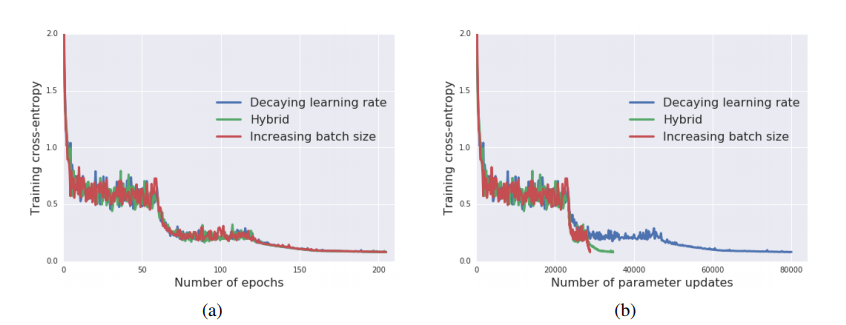• So what is a batch size in NN (another source) - and how to find the “right” number. In general terms a good mini bach between 1 and all samples is a good idea. Figure it out empirically.
• one epoch = one forward pass and one backward pass of all the training examples
• batch size = the number of training examples in one forward/backward pass. The higher the batch size, the more memory space you'll need.
• number of iterations = number of passes, each pass using [batch size] number of examples. To be clear, one pass = one forward pass + one backward pass (we do not count the forward pass and backward pass as two different passes).
Example: if you have 1000 training examples, and your batch size is 500, then it will take 2 iterations to complete 1 epoch.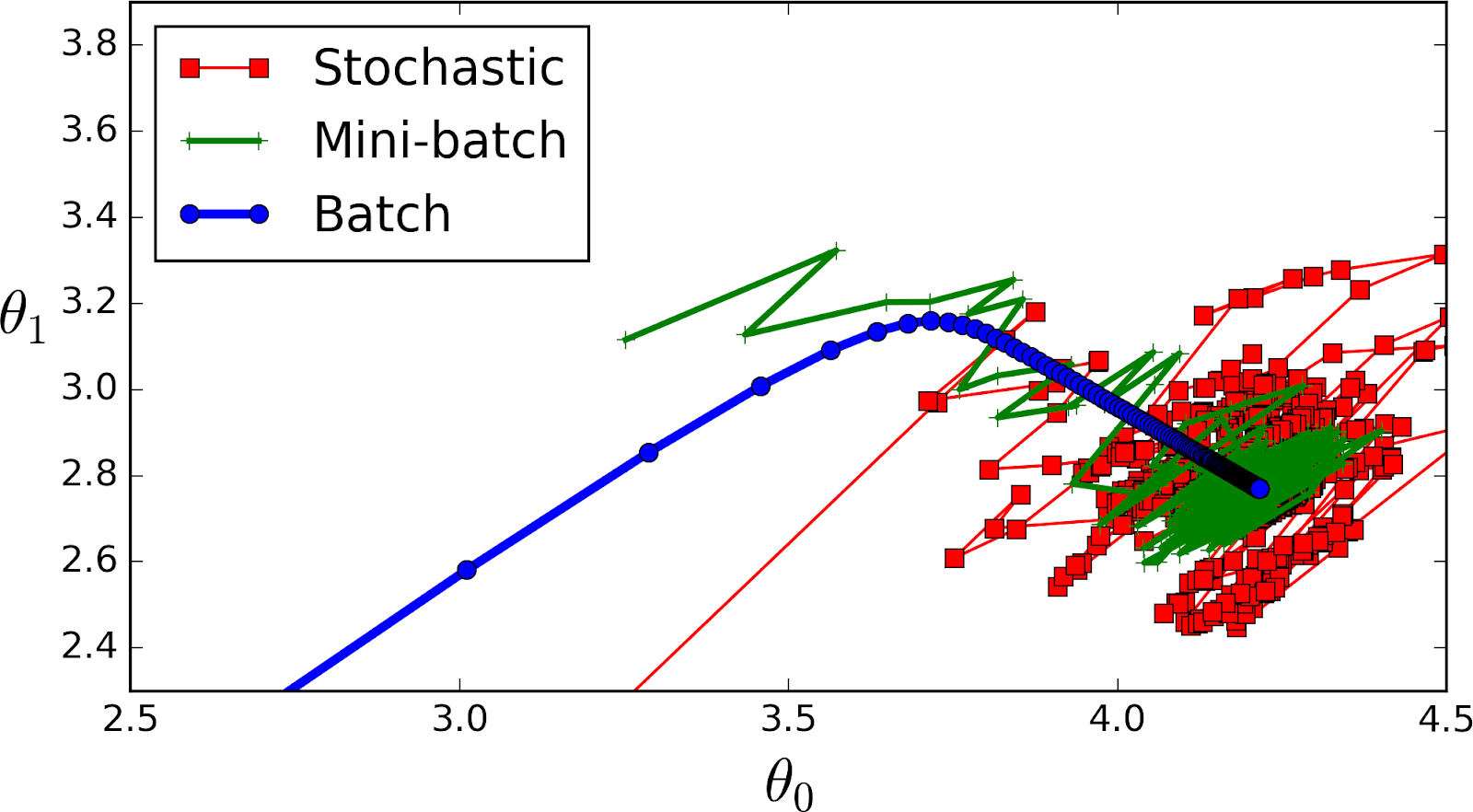GD with Momentum - explain

## Batch size

A sequence prediction problem makes a good case for a varied batch size as you may want to have a batch size equal to the training dataset size (batch learning) during training and a batch size of 1 when making predictions for one-step outputs.
power of 2: have some advantages with regards to vectorized operations in certain packages, so if it's close it might be faster to keep your batch_size in a power of 2.
Batch size defines number of samples that going to be propagated through the network.
For instance, let's say you have 1050 training samples and you want to set up batch_size equal to 100. Algorithm takes first 100 samples (from 1st to 100th) from the training dataset and trains network. Next it takes second 100 samples (from 101st to 200th) and train network again. We can keep doing this procedure until we will propagate through the networks all samples. The problem usually happens with the last set of samples. In our example we've used 1050 which is not divisible by 100 without remainder. The simplest solution is just to get final 50 samples and train the network.
• It requires less memory. Since you train network using less number of samples the overall training procedure requires less memory. It's especially important in case if you are not able to fit dataset in memory.
• Typically networks trains faster with mini-batches. That's because we update weights after each propagation. In our example we've propagated 11 batches (10 of them had 100 samples and 1 had 50 samples) and after each of them we've updated network's parameters. If we used all samples during propagation we would make only 1 update for the network's parameter.
• The smaller the batch the less accurate estimate of the gradient. In the figure below you can see that mini-batch (green color) gradient's direction fluctuates compare to the full batch (blue color).enter image description here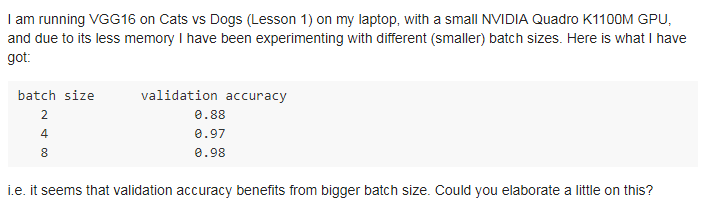IMPORTANT: batch size in ‘.prediction’ is needed for some models, only for technical reasons as seen here, in keras.
1. 1.
2. 2.
Another observation, probably empirical - to answer your questions on Batch Size and Epochs:
In general: Larger batch sizes result in faster progress in training, but don't always converge as fast. Smaller batch sizes train slower, but can converge faster. It's definitely problem dependent.
In general, the models improve with more epochs of training, to a point. They'll start to plateau in accuracy as they converge. Try something like 50 and plot number of epochs (x axis) vs. accuracy (y axis). You'll see where it levels out.

## BIAS

The role of bias in NN - similarly to the ‘b’ in linear regression.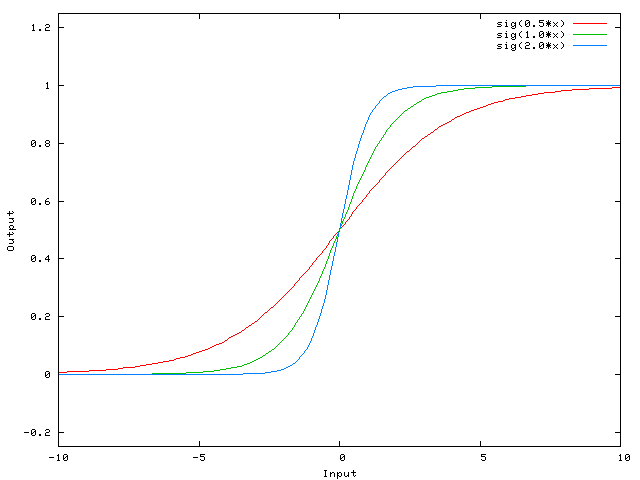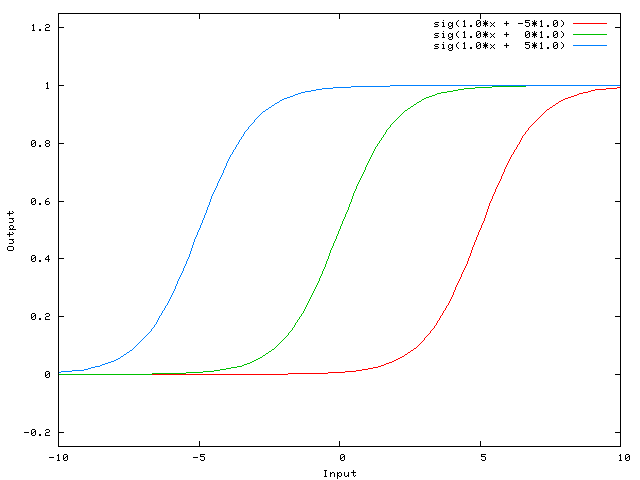## BATCH NORMALIZATION

1. 1.
The best explanation to what is BN and why to use it, including busting the myth that it solves internal covariance shift - shifting input distribution, and saying that it should come after activations as it makes more sense (it does),also a nice quote on where a layer ends is really good - it can end at the activation (or not). How to use BN in the test, hint: use a moving window. Bn allows us to use 2 parameters to control the input distribution instead of controlling all the weights.
2. 2.
3. 3.
• Layer normalization (Ba 2016): Does not use batch statistics. Normalize using the statistics collected from all units within a layer of the current sample. Does not work well with ConvNets.
• Recurrent Batch Normalization (BN) (Cooijmans, 2016; also proposed concurrently by Qianli Liao & Tomaso Poggio, but tested on Recurrent ConvNets, instead of RNN/LSTM): Same as batch normalization. Use different normalization statistics for each time step. You need to store a set of mean and standard deviation for each time step.
• Batch Normalized Recurrent Neural Networks (Laurent, 2015): batch normalization is only applied between the input and hidden state, but not between hidden states. i.e., normalization is not applied over time.
• Streaming Normalization (Liao et al. 2016) : it summarizes existing normalizations and overcomes most issues mentioned above. It works well with ConvNets, recurrent learning and online learning (i.e., small mini-batch or one sample at a time):
• Weight Normalization (Salimans and Kingma 2016): whenever a weight is used, it is divided by its L2 norm first, such that the resulting weight has L2 norm 1. That is, output y=x∗(w/|w|), where x and w denote the input and weight respectively. A scalar scaling factor g is then multiplied to the output y=y∗g. But in my experience g seems not essential for performance (also downstream learnable layers can learn this anyway).
• Cosine Normalization (Luo et al. 2017): weight normalization is very similar to cosine normalization, where the same L2 normalization is applied to both weight and input: y=(x/|x|)∗(w/|w|). Again, manual or automatic differentiation can compute appropriate gradients of x and w.
• Note that both Weight and Cosine Normalization have been extensively used (called normalized dot product) in the 2000s in a class of ConvNets called HMAX (Riesenhuber 1999) to model biological vision. You may find them interesting.
1. 1.
Layer normalization solves the rnn case that batch couldnt - Is done per feature within the layer and normalized features are replaced
2. 2.
Instance does it for (cnn?) using per channel normalization
3. 3.
Group does it for group of channels
4. 4.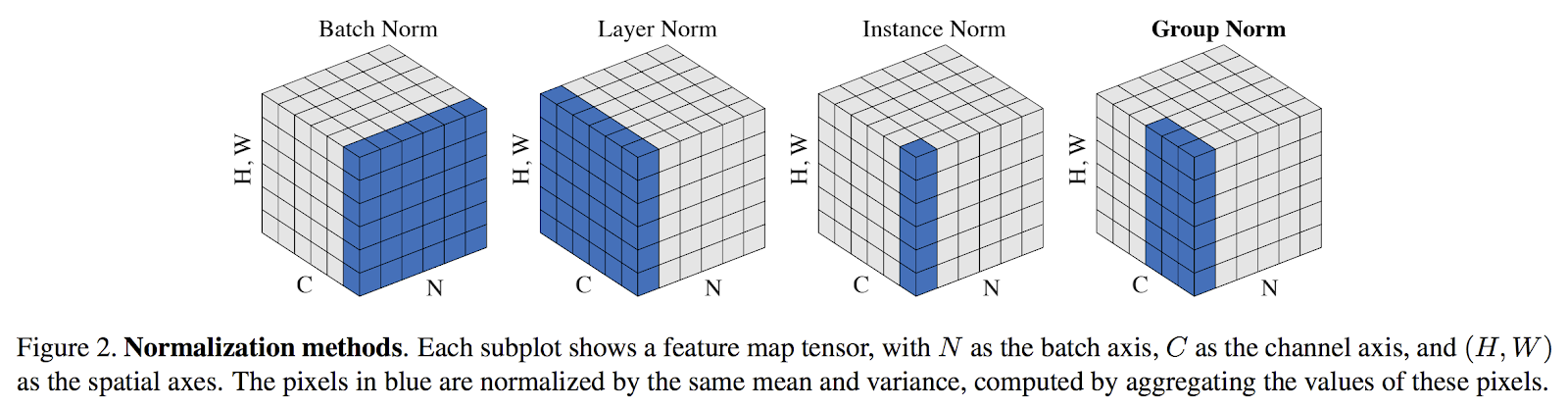Part2: batch/layer/weight normalization - This is a good resource for advantages for every layer
• Layer, per feature in a batch,
• weight - divided by the norm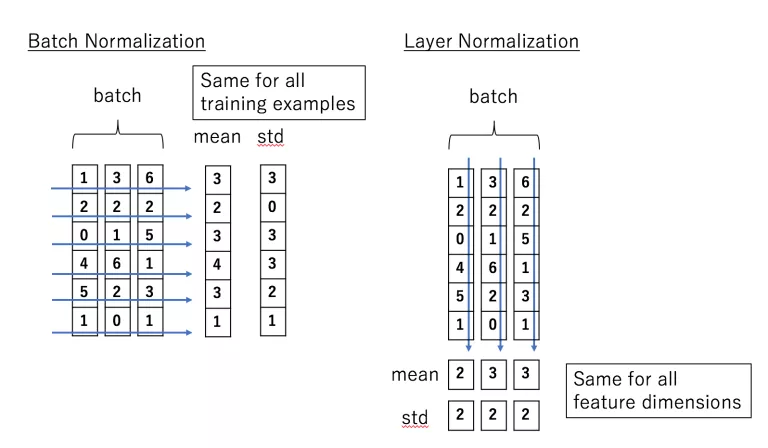## LOSS

Very Basic advice: You should probably switch train/validation repartition to something like 80% training and 20% validation. In most cases it will improve the classifier performance overall (more training data = better performance)
+If Training error and test error are too close (your system is unable to overfit on your training data), this means that your model is too simple. Solution: more layers or more neurons per layer.
Early stopping
If you have never heard about "early-stopping" you should look it up, it's an important concept in the neural network domain : https://en.wikipedia.org/wiki/Early_stopping . To summarize, the idea behind early-stopping is to stop the training once the validation loss starts plateauing. Indeed, when this happens it almost always mean you are starting to overfitt your classifier. The training loss value in itself is not something you should trust, beacause it will continue to increase event when you are overfitting your classifier.
With cross entropy there can be an issue where the accuracy is the same for two cases, one where the loss is decreasing and the other when the loss is not changing much.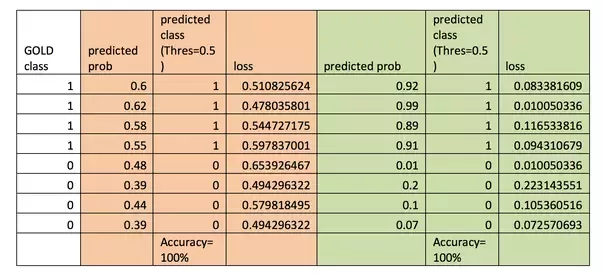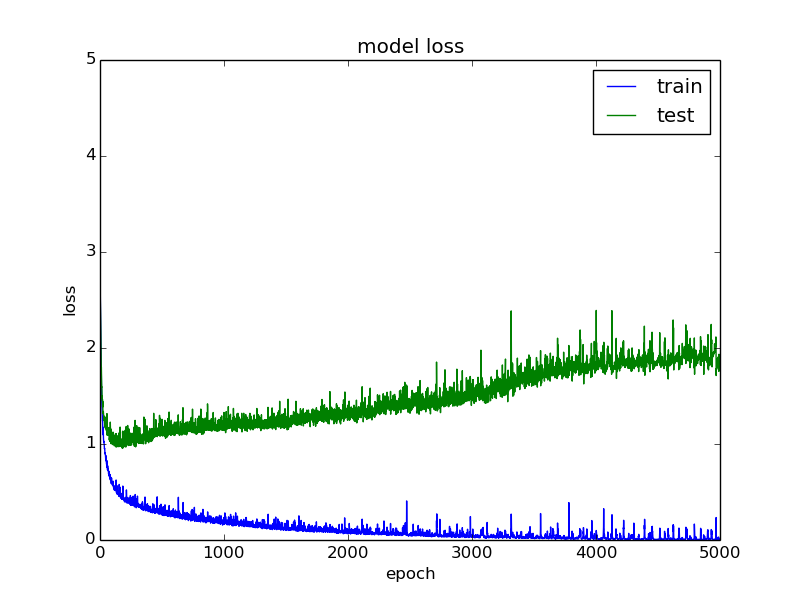This indicates that the model is overfitting. It continues to get better and better at fitting the data that it sees (training data) while getting worse and worse at fitting the data that it does not see (validation data).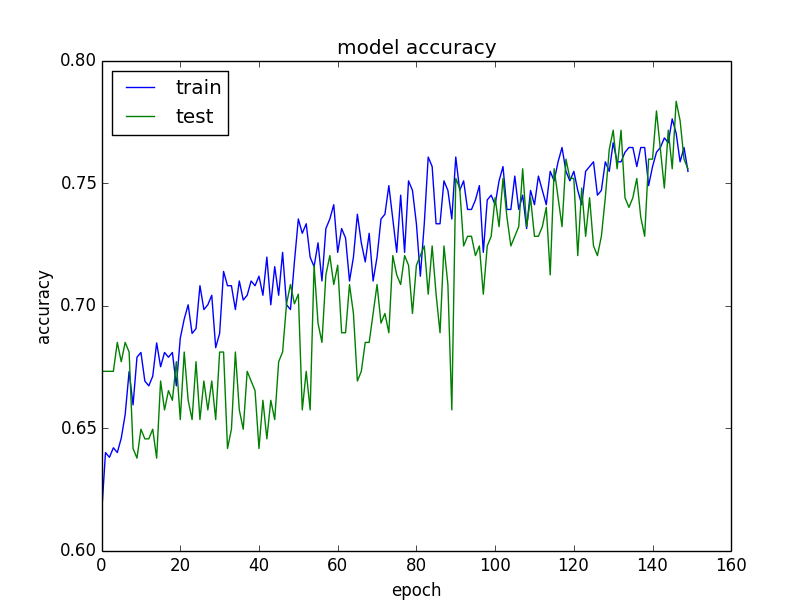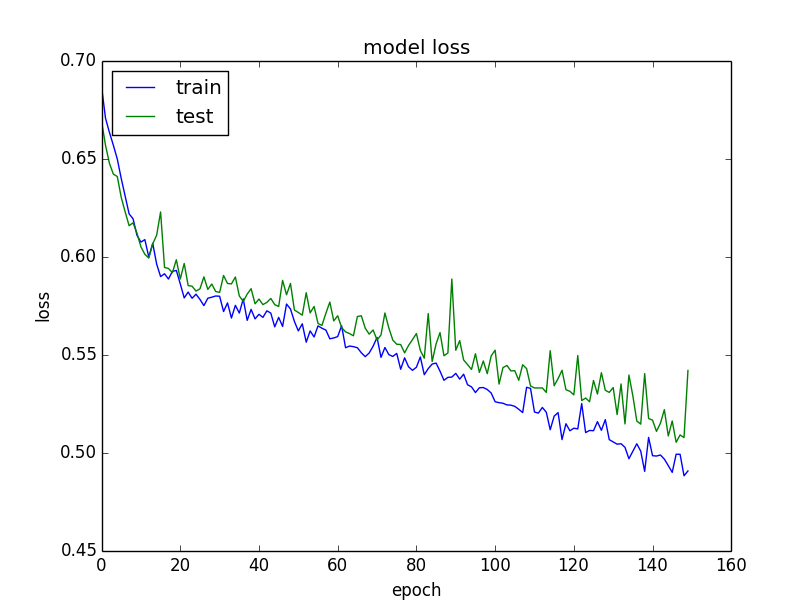## LEARNING RATE REDUCTION

Intro to Learning Rate methods - what they are doing and what they are fixing in other algos.
Callbacks, especially ReduceLROnPlateau - this callback monitors a quantity and if no improvement is seen for a 'patience' number of epochs, the learning rate is reduced.
Cs123 (very good): explains about many things related to CNN, but also about LR and adaptive methods.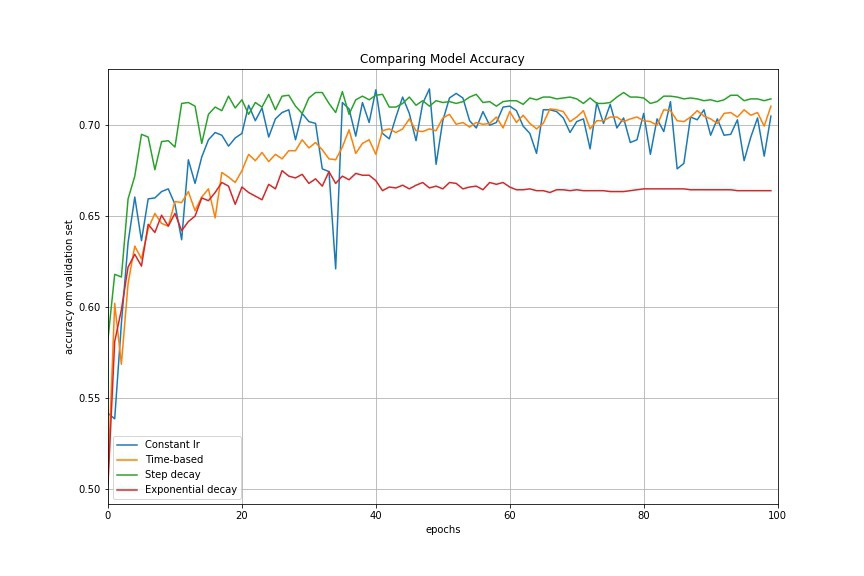These per-parameter learning rate methods provide heuristic approach without requiring expensive work in tuning hyperparameters for the learning rate schedule manually.
1. 1.
Adagrad performs larger updates for more sparse parameters and smaller updates for less sparse parameter. It has good performance with sparse data and training large-scale neural network. However, its monotonic learning rate usually proves too aggressive and stops learning too early when training deep neural networks.
2. 2.
3. 3.
RMSprop adjusts the Adagrad method in a very simple way in an attempt to reduce its aggressive, monotonically decreasing learning rate.
4. 4.
Adam is an update to the RMSProp optimizer which is like RMSprop with momentum.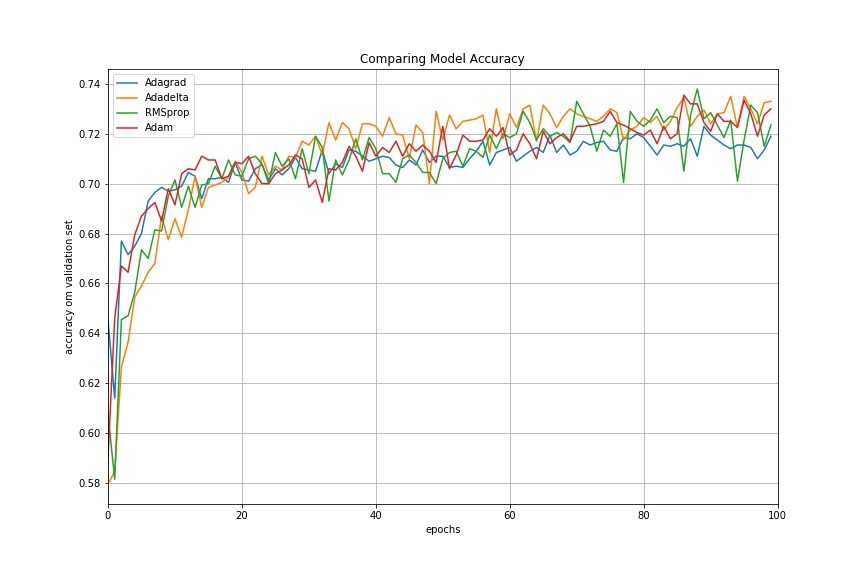adaptive learning rate methods demonstrate better performance than learning rate schedules, and they require much less effort in hyperparamater settings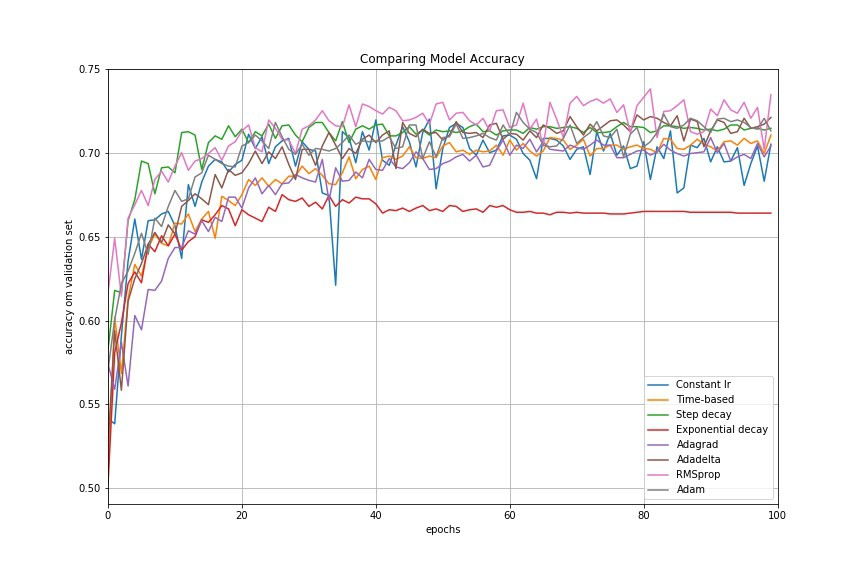Recommended paper: practical recommendation for gradient based DNN
Another great comparison - pdf paper and webpage link -
• if your input data is sparse, then you likely achieve the best results using one of the adaptive learning-rate methods.
• An additional benefit is that you will not need to tune the learning rate but will likely achieve the best results with the default value.

## TRAIN / VAL accuracy in NN

The second important quantity to track while training a classifier is the validation/training accuracy. This plot can give you valuable insights into the amount of overfitting in your model: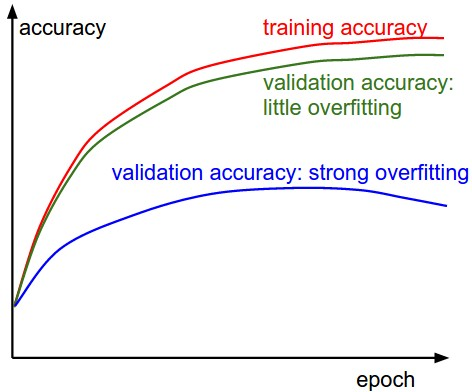• The gap between the training and validation accuracy indicates the amount of overfitting.
• Two possible cases are shown in the diagram on the left. The blue validation error curve shows very small validation accuracy compared to the training accuracy, indicating strong overfitting (note, it's possible for the validation accuracy to even start to go down after some point).
• NOTE: When you see this in practice you probably want to increase regularization:
• stronger L2 weight penalty
• Dropout
• collect more data.
• The other possible case is when the validation accuracy tracks the training accuracy fairly well. This case indicates that your model capacity is not high enough: make the model larger by increasing the number of parameters.

## INITIALIZERS

XAVIER GLOROT:
In short, it helps signals reach deep into the network.
• If the weights in a network start too small, then the signal shrinks as it passes through each layer until it’s too tiny to be useful.
• If the weights in a network start too large, then the signal grows as it passes through each layer until it’s too massive to be useful.
Xavier initialization makes sure the weights are ‘just right’, keeping the signal in a reasonable range of values through many layers.
To go any further than this, you’re going to need a small amount of statistics - specifically you need to know about random distributions and their variance.
However, i am still not seeing anything empirical that says that glorot surpesses everything else under certain conditions (except the glorot paper), most importantly, does it really help in LSTM where the vanishing gradient is ~no longer an issue?
This method of initializing became famous through a paper submitted in 2015 by He et al, and is similar to Xavier initialization, with the factor multiplied by two. In this method, the weights are initialized keeping in mind the size of the previous layer which helps in attaining a global minimum of the cost function faster and more efficiently.
w=np.random.randn(layer_size[l],layer_size[l-1])*np.sqrt(2/layer_size[l-1])

## ACTIVATION FUNCTIONS

1. 1.
1. 1.
Output layer - linear for regression, softmax for classification
2. 2.
Hidden layers - hyperbolic tangent for shallow networks (less than 3 hidden layers), and ReLU for deep networks
2. 2.
ReLU - The purpose of ReLU is to introduce non-linearity, since most of the real-world data we would want our network to learn would be nonlinear (e.g. convolution is a linear operation – element wise matrix multiplication and addition, so we account for nonlinearity by introducing a nonlinear function like ReLU, e.g here - search for ReLU).
1. 1.
Relu is quite resistant to vanishing gradient & allows for deactivating neurons and for sparsity.
2. 2.
Other nonlinear functions such as tanh or sigmoid can also be used instead of ReLU, but ReLU has been found to perform better in most situations.
3. 5.
Selu - better than RELU? Possibly.
4. 6.
Mish: A Self Regularized Non-Monotonic Neural Activation Function, yam peleg’s code
5. 8.
Gelu (Used by OpenAI

## OPTIMIZERS

There are several optimizers, each had his 15 minutes of fame, some optimizers are recommended for CNN, Time Series, etc..
There are also what I call ‘experimental’ optimizers, it seems like these pop every now and then, with or without a formal proof. It is recommended to follow the literature and see what are the ‘supposedly’ state of the art optimizers atm.
Backstitch - September 17 - supposedly an improvement over SGD for speech recognition using DNN. Note: it wasnt tested with other datasets or other network types.
(how does it work?) take a negative step back, then a positive step forward. I.e., When processing a minibatch, instead of taking a single SGD step, we first take a step with −α times the current learning rate, for α > 0 (e.g. α = 0.3), and then a step with 1 + α times the learning rate, with the same minibatch (and a recomputed gradient). So we are taking a small negative step, and then a larger positive step. This resulted in quite large improvements – around 10% relative improvement  – for our best speech recognition DNNs. The recommended hyper parameters are in the paper.
Drawbacks: takes twice to train, momentum not implemented or tested, dropout is mandatory for improvement, slow starter.
• SGD can be fine tuned
• For others Leave most parameters as they were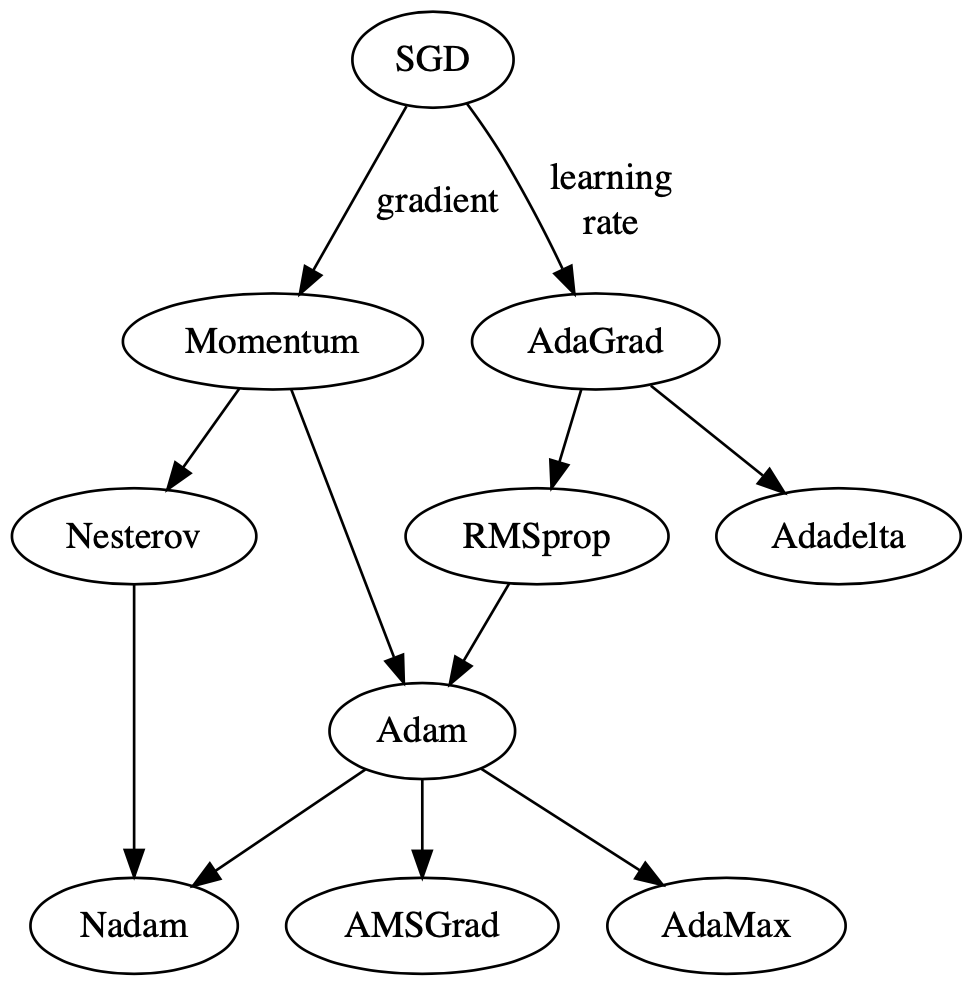## DROPOUT LAYERS IN KERAS AND GENERAL

OPEN QUESTIONs:
1. 1.
does a dropout layer improve performance even if an lstm layer has dropout or recurrent dropout.
2. 2.
What is the diff between a separate layer and inside the lstm layer.
3. 3.
What is the diff in practice and intuitively between drop and recurrentdrop
• Dropout is a technique where randomly selected neurons are ignored RANDOMLY during training.
• contribution to the activation of downstream neurons is temporally removed on the forward pass and any weight updates are not applied to the neuron on the backward pass.
• As a neural network learns, neuron weights settle into their context within the network.
• Weights of neurons are tuned for specific features providing some specialization. Neighboring neurons become to rely on this specialization, which if taken too far can result in a fragile model too specialized to the training data. (overfitting)
• This reliant on context for a neuron during training is referred to complex co-adaptations.
• After dropout, other neurons will have to step in and handle the representation required to make predictions for the missing neurons, which is believed to result in multiple independent internal representations being learned by the network.
• Thus, the effect of dropout is that the network becomes less sensitive to the specific weights of neurons.
• This in turn leads to a network with better generalization capability and less likely to overfit the training data.
• as a consequence of the 50% dropout, the neural network will learn different, redundant representations; the network can’t rely on the particular neurons and the combination (or interaction) of these to be present.
• Another nice side effect is that training will be faster.
• Rules:
• Dropout is only applied during training,
• Need to rescale the remaining neuron activations. E.g., if you set 50% of the activations in a given layer to zero, you need to scale up the remaining ones by a factor of 2.
• if the training has finished, you’d use the complete network for testing (or in other words, you set the dropout probability to 0).
Implementation of drop out in keras is “inverse dropout” - n the Keras implementation, the output values are corrected during training (by dividing, in addition to randomly dropping out the values) instead of during testing (by multiplying). This is called "inverted dropout".
Inverted dropout is functionally equivalent to original dropout (as per your link to Srivastava's paper), with a nice feature that the network does not use dropout layers at all during test and prediction. This is explained a little in this Keras issue.
• dropout value of 20%-50% of neurons with 20% providing a good starting point. (A probability too low has minimal effect and a value too high results in underlearning by the network.)
• Use a large network for better performance, i.e., when dropout is used on a larger network, giving the model more of an opportunity to learn independent representations.
• Use dropout on VISIBLE AND HIDDEN. Application of dropout at each layer of the network has shown good results.
• Unclear ? Use a large learning rate with decay and a large momentum. Increase your learning rate by a factor of 10 to 100 and use a high momentum value of 0.9 or 0.99.
• Unclear ? Constrain the size of network weights. A large learning rate can result in very large network weights. Imposing a constraint on the size of network weights such as max-norm regularization with a size of 4 or 5 has been shown to improve results.
I suggest taking a look at (the first part of) this paper. Regular dropout is applied on the inputs and/or the outputs, meaning the vertical arrows from x_t and to h_t. In you add it as an argument to your layer, it will mask the inputs; you can add a Dropout layer after your recurrent layer to mask the outputs as well. Recurrent dropout masks (or "drops") the connections between the recurrent units; that would be the horizontal arrows in your picture.
This picture is taken from the paper above. On the left, regular dropout on inputs and outputs. On the right, regular dropout PLUS recurrent dropout: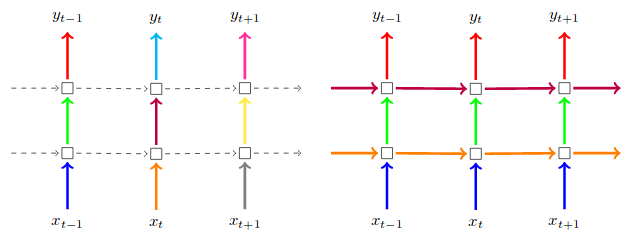This picture is taken from the paper above. On the left, regular dropout on inputs and outputs. On the right, regular dropout PLUS recurrent dropout.

## NEURAL NETWORK OPTIMIZATION TECHNIQUES

Basically do these after you have a working network
1. 3.
****RESNET, DENSENET UNET - the trick behind them, concatenating both f(x) = x
2. 4.
****skip connections **** by Siravam / Vidhya- **"**Skip Connections (or Shortcut Connections) as the name suggests skips some of the layers in the neural network and feeds the output of one layer as the input to the next layers.
Skip Connections were introduced to solve different problems in different architectures. In the case of ResNets, skip connections solved the degradation problem that we addressed earlier whereas, in the case of DenseNets, it ensured feature reusability. We’ll discuss them in detail in the following sections.
Skip connections were introduced in literature even before residual networks. For example, Highway Networks (Srivastava et al.) had skip connections with gates that controlled and learned the flow of information to deeper layers. This concept is similar to the gating mechanism in LSTM. Although ResNets is actually a special case of Highway networks, the performance isn’t up to the mark comparing to ResNets. This suggests that it’s better to keep the gradient highways clear than to go for any gates – simplicity wins here!"

## Deep Learning for NLP

• (did not fully read) Yoav Goldberg’s course syllabus with lots of relevant topics on DL4NLP, including bidirectional RNNS and tree RNNs.
• (did not fully read) CS224d: Deep Learning for Natural Language Processing, with slides etc.
Deep Learning using Linear Support Vector Machines - 1-3% decrease in error by replacing the softmax layer with a linear support vector machine

## MULTI LABEL/OUTPUT

1. 1.
A machine learning framework for multi-output/multi-label and stream data. Inspired by MOA and MEKA, following scikit-learn's philosophy. https://scikit-multiflow.github.io/

## SIAMESE NETWORKS

1. 2.
Burlow, fb post - Self-supervised learning (SSL) is rapidly closing the gap with supervised methods on large computer vision benchmarks. A successful approach to SSL is to learn representations which are invariant to distortions of the input sample. However, a recurring issue with this approach is the existence of trivial constant solutions. Most current methods avoid such solutions by careful implementation details. We propose an objective function that naturally avoids such collapse by measuring the cross-correlation matrix between the outputs of two identical networks fed with distorted versions of a sample, and making it as close to the identity matrix as possible. This causes the representation vectors of distorted versions of a sample to be similar, while minimizing the redundancy between the components of these vectors.

## Gated Multi-Layer Perceptron (GMLP)

1. 1.
****paper, git1, git2 **- "**a simple network architecture, gMLP, based on MLPs with gating, and show that it can perform as well as Transformers in key language and vision applications. Our comparisons show that self-attention is not critical for Vision Transformers, as gMLP can achieve the same accuracy."